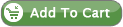Search By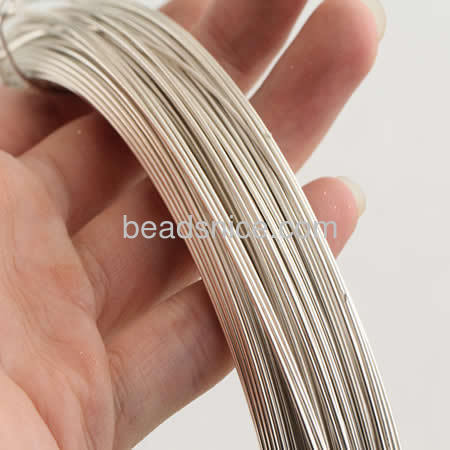28 Gauge sterling silver wire, 0.3mm, sold by M, ID 26880
ID26880
Weight1 gram
Size0.3mm
Min.Order 50 M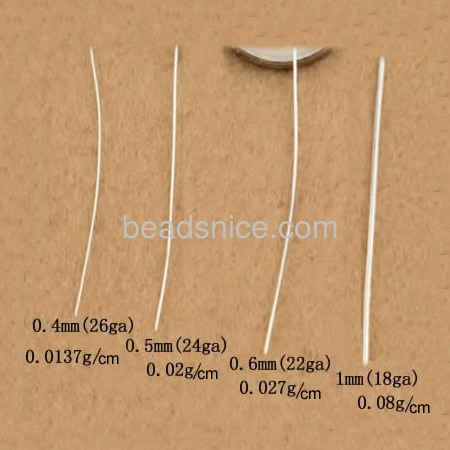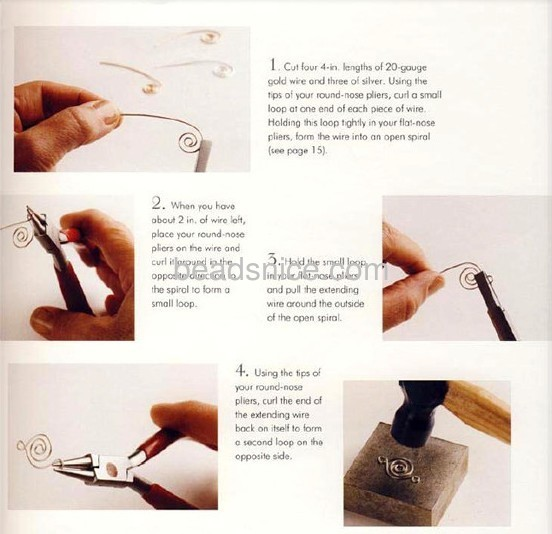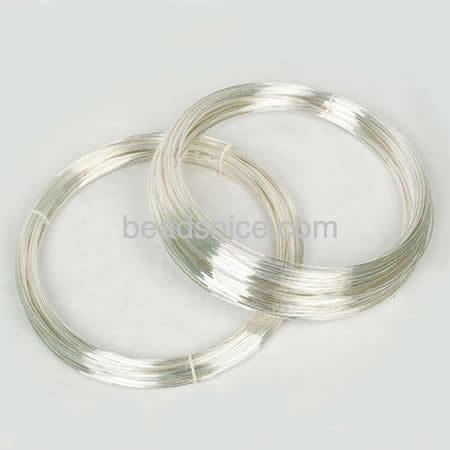Description:
14 gauge = 1.6 mm = 0.06" 14 inches = 1.17 feet = 0.35 meters 16 gauge = 1.3 mm = 0.5" 22.5 inches = 1.87 feet = 0.57 meters 18 gauge = 1 mm = 0.039" 3 feet = 0.91 meters 19 gauge = .9 mm = 0.036" 3.75 feet = 1.14 meters 20 gauge = .8 mm = 0.031" 4.75 feet = 1.44 meters 22 gauge = .64mm = 0.025" 7.63 feet = 2.32 meter 24 gauge = .5 mm = 0.019" 12 feet = 3.65 meters
If you choose the mix-color option,we will pick out the colors which are available in our current stock.Subtotal: \$